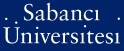# Linear complexity over F-q and over F-qm for linear recurring sequences

Meidl, Wilfried and Özbudak, Ferruh (2009) Linear complexity over F-q and over F-qm for linear recurring sequences. Finite Fields and Their Applications, 15 (1). pp. 110-124. ISSN 1071-5797

This is the latest version of this item.Preview
PDF - Requires a PDF viewer such as GSview, Xpdf or Adobe Acrobat Reader
244Kb

Official URL: http://dx.doi.org/10.1016/j.ffa.2008.09.004

## Abstract

Since the F-q-linear spaces F-q(m) and F-qm are isomorphic, an m-fold multisequence S over the finite field F-q with a given characteristic polynomial f is an element of F-q[x], can be identified with a single sequence S over F-qm with characteristic polynomial f. The linear complexity of S, which will be called the generalized joint linear complexity of S, can be significantly smaller than the conventional joint linear complexity of S. We determine the expected value and the variance of the generalized joint linear complexity of a random m-fold multisequence S with given minimal polynomial. The result on the expected value generalizes a previous result oil periodic m-fold multisequences. Moreover we determine the expected drop of linear complexity of a random m-fold multisequence with given characteristic polynomial f, when one switches from conventional joint linear complexity to generalized joint linear complexity.

Item Type: Article Joint linear complexity; Generalized joint linear complexity; Multisequences; Linear recurring sequences UNSPECIFIED 11480 Wilfried Meidl 29 Apr 2009 10:21 25 May 2011 14:07

### Available Versions of this Item

Repository Staff Only: item control page